Math Courses / Course / Chapter

# What Is Equivalent in Math?

Roxanne Comegys, Bethany Calderwood, Laura Pennington
• Author
Roxanne Comegys

Dr. Comegys has taught high school math for 11 years including Math 1, Coordinate Algebra, Algebra 1, Geometry, Advanced Algebra, Algebra 2, Math 3, Pre Calculus, and AP Calculus. She has earned a B.A. in Educational Studies from Emory University as well as a M.A.T. in Middle Grades Math and a Ph. D. in Curriculum and Instruction both from Mercer University. She has teaching certificate in math for middle and secondary grades and holds a STEM endorsement. She has a service certificate in curriculum and instruction.

• Instructor
Bethany Calderwood

Bethany is a certified Special Education and Elementary teacher with 11 years experience teaching Special Education from grades PK through 5. She has a Bachelor's degree in Special Education, Elementary Education, and English from Gordon College and a Master's degree in Special Education from Salem State University.

• Expert Contributor
Laura Pennington

Laura received her Master's degree in Pure Mathematics from Michigan State University, and her Bachelor's degree in Mathematics from Grand Valley State University. She has 20 years of experience teaching collegiate mathematics at various institutions.

What does equivalent mean in math? Learn the equivalent definitions, equivalent numbers, and equivalent fractions. Learn equivalent with the help of examples. Updated: 11/30/2021

Show
• FAQs
• Activities

## Equivalent Numbers Bingo Game

### Reminder:

• Equivalent numbers are numbers that have the same value.
• Each type of number, such as fractions, decimals, or square roots, can be equivalent to other numbers of their types, or to numbers of different types, as long as they have the same value.

### Materials Needed:

• Bingo cards with various numbers on them in a rectangular pattern
• Bingo chips
• A bucket or container of numbers on pieces of paper, such that the numbers on the bingo cards are equivalent to these numbers.

### Directions and Rules:

1. Hand out bingo cards and bingo chips to each player. This can be played alone or with a group. However many bingo cards you have is the number of people that can play.
2. Pull a number out of the number bucket.
3. Each player places a bingo chip on any number on their card that is equivalent to the number pulled from the bucket.
4. This continues until someone gets a row, column, or diagonal of chips on their card. At this point, the person with a full row, column, or diagonal says "equivalent numbers bingo".
5. Whoever gets a correct equivalent numbers bingo first wins the game.

### Sample Round of One Number Being Pulled From the Number Bucket

Gary and Fiona are playing equivalent numbers bingo. The first number pulled from the bucket is 0.2. Fiona sees that she has the number √(0.04) and the number 2/10 on her card. First, she looks at √(0.04). She knows that 22 = 4, so she sees a possible connection between 0.2 and √(0.04), and decides to calculate √(0.04).

• √(0.04) = 0.2

Sure enough, she finds that √(0.04) = 0.2, so she knows that these two numbers are equivalent, and she places a chip over √(0.04). She then considers the number 0.2 as a fraction. Since the 2 is in the tenths place, the number is read as two-tenths, so she knows that the fraction, 2/10, is equivalent to 0.2, so she places a chip over that number as well.

Gary is looking at his card, and doesn't see anything that looks equivalent to 0.2, but he knows that if he performs the division indicated by the fractions on his card, then he could find that they actually do have the same value as 0.2. There are two fractions on his card; 3/8 and 1/5, so he performs the division for each of these.

• 3 ÷ 8 = 0.375
• 1 ÷ 5 = 0.2

He sees that 3/8 has a decimal value of 0.375, so this is not equivalent to 0.2. However, he also sees that 1/5 has a decimal value of 0.2, so this is equivalent to 0.2, and he places a chip over 1/5. After this, the next number is pulled from the bucket, and they play until one of them gets an equivalence number bingo.

#### What does the word "equivalent" in math mean?

Equivalent in math refers to equality. It means that two numbers represent the same value or ratio. Values can be written as equivalent fractions, decimals, percentages, or whole numbers.

#### What does equivalent fractions mean in math?

Equivalent fractions refer to fractions that represent the same ratio. Examples of equivalent fractions include 2/3 and 4/6. Equivalent fractions can be created by multiplying the numerator and denominator by the same factor. Two or more fractions can be verified as equivalent by trying to find a common factor between the numerators and denominators of the two fractions.

The word equivalent in math describes when things are equal in value. For example, if two students have the same number of treats, then the amount of treats that one student has is equivalent to the amount that another one has.

### Equivalent Definition

Equivalent: When two or more quantities have the same value. An equal sign is used to show that the quantities are the same value.

### What is equivalent?

The quantities can be represented by a number, fraction, decimal, or percentage. Equivalent does not always mean that the values look identical.

### Equivalent Numbers

Numbers can be used to represent values in different ways. The different representations are equivalent to each other. They look different but represent the same value. They can take on different forms and can be changed from one form to another. When the value changes, it is referred to it as converting. The lesson will discuss the idea of representing values using different types of numbers as fractions, decimals, and percentages.

### Equivalent Fractions

Fractions are equivalent to one another when they represent the same ratio or value. A fraction has a numerator and a denominator. The denominator describes the number of parts 1 whole has been split into. The numerator describes how many of those parts are being used. For example, the fraction 1/2 shows that a whole was broken into two parts, and one of those parts is being used. This relationship is referred to as a ratio. For example, in the case of 1/2, it shows that one-half of the whole thing was used. In this case, 1/2, a whole is being broken into two parts, and one of those parts is being used. The same ratio occurs in the case of 2/4. The whole has been broken into four parts, and two of those parts have been used. Since 2 is half of 4, then 2/4 represents the same ratio as 1/2. So 1/2 and 2/4 are equivalents. Although they have different numbers, the fractions represent the same value. So, they are equivalent.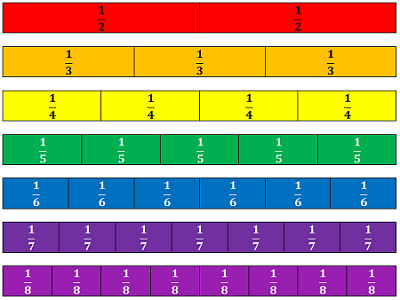Also, if two fractions are equivalent, the product of the numerator of the first fraction and the denominators of the second fraction should be the same as the product of the denominator of the first fraction and the numerator of the second fraction. This is called cross-multiplication. It can be used to verify that fractions are equivalent to each other and show the same ratio. In the example below, the two products would be equal since 21 x 5 = 105 and 3 x 35 =105. So, the fractions are equivalent to each other.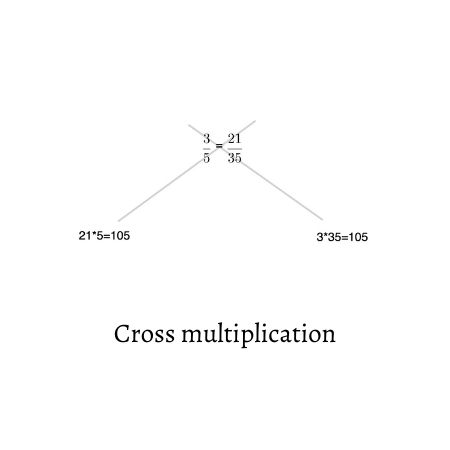To unlock this lesson you must be a Study.com Member.

## Equal Pieces of Cake

Mom made mini cakes for dessert. She gave Chris one-half of a cake, Leila three-sixths of a cake, and Jim four-eighths of a cake. Chris said it wasn't fair because Jim got four pieces of cake, while he only got one. Leila said it wasn't fair because Chris' half of the cake was bigger than her three pieces. Jim said he thought all their portions were the same. Who was right?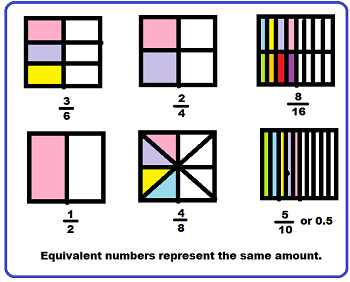Look at this picture. One half, three-sixths, and four-eighths are all fractions that show the same amount of cake! The picture shows that two-fourths, eight-sixteenths, and five-tenths are also equal to the same amount of cake. This is because all of the portions are equivalent fractions.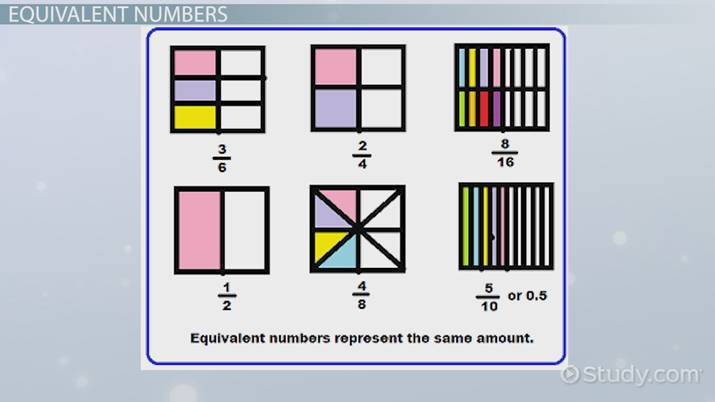An error occurred trying to load this video.

Try refreshing the page, or contact customer support.

Coming up next: 1st Grade Math Vocabulary: Lesson for Kids

### You're on a roll. Keep up the good work!

Replay
Your next lesson will play in 10 seconds
• 0:03 Equal Pieces of Cake
• 0:46 Equivalent Numbers
• 2:29 Converting Decimals…
• 3:33 Lesson Summary
Save Timeline
Autoplay
Autoplay
Speed Speed

Equivalent refers to numbers that are different representations of the same value. This means that they are equal to each other. Equivalent numbers can be rewritten as fractions. decimals, or percentages. A factor can be used to create equivalent forms of numbers. Fractions, decimals, and percentages are used to describe different versions of the same ratio and can be used to show equivalent forms of numbers. Percentages can also be represented as decimals.

To unlock this lesson you must be a Study.com Member.

## Equivalent Numbers

Equivalent means equal in value, function, or meaning. In math, equivalent numbers are numbers that are written differently but represent the same amount. In the picture of the boxes, the same amount of each box is colored in, but because each box is divided into a different number of sections, the number is written differently.

### Equivalent Fractions

Equivalent fractions can be found by multiplying both the numerator (top number) and denominator (bottom number) by the same number. They can also be found by reducing a fraction to its simplest terms.

In order to add or subtract fractions, they need to have like denominators. That means the fractions must have the same number on the bottom of each fraction. To add or subtract fractions, it's often necessary to use equivalent fractions. Look at this problem.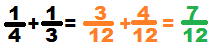As they stand now, the fractions 1/4 and 1/3 cannot be added, but as equivalent fractions of 3/12 and 4/12, they can be added.

### Equivalent Decimals

When you're working with decimals, sometimes it is helpful to add a zero as a placeholder. This is okay if the zero is placed to the right of the decimal point after the last number. A decimal and a zero can also be added after a whole number, which is often done to facilitate long division and multiplication. Here are a few examples of equivalent decimals, or decimals that have the same value:

• 3.4 = 3.40
• 5 = 5.0
• 2.62 = 2.620

These are not equivalent because adding a zero changes the value of the number:

• 3.4 and 3.04
• 9.4 and 90.4
• 4.55 and 4.505
Video Transcript

## Equal Pieces of Cake

Mom made mini cakes for dessert. She gave Chris one-half of a cake, Leila three-sixths of a cake, and Jim four-eighths of a cake. Chris said it wasn't fair because Jim got four pieces of cake, while he only got one. Leila said it wasn't fair because Chris' half of the cake was bigger than her three pieces. Jim said he thought all their portions were the same. Who was right?Look at this picture. One half, three-sixths, and four-eighths are all fractions that show the same amount of cake! The picture shows that two-fourths, eight-sixteenths, and five-tenths are also equal to the same amount of cake. This is because all of the portions are equivalent fractions.

## Equivalent Numbers

Equivalent means equal in value, function, or meaning. In math, equivalent numbers are numbers that are written differently but represent the same amount. In the picture of the boxes, the same amount of each box is colored in, but because each box is divided into a different number of sections, the number is written differently.

### Equivalent Fractions

Equivalent fractions can be found by multiplying both the numerator (top number) and denominator (bottom number) by the same number. They can also be found by reducing a fraction to its simplest terms.

In order to add or subtract fractions, they need to have like denominators. That means the fractions must have the same number on the bottom of each fraction. To add or subtract fractions, it's often necessary to use equivalent fractions. Look at this problem.As they stand now, the fractions 1/4 and 1/3 cannot be added, but as equivalent fractions of 3/12 and 4/12, they can be added.

### Equivalent Decimals

When you're working with decimals, sometimes it is helpful to add a zero as a placeholder. This is okay if the zero is placed to the right of the decimal point after the last number. A decimal and a zero can also be added after a whole number, which is often done to facilitate long division and multiplication. Here are a few examples of equivalent decimals, or decimals that have the same value:

• 3.4 = 3.40
• 5 = 5.0
• 2.62 = 2.620

These are not equivalent because adding a zero changes the value of the number:

• 3.4 and 3.04
• 9.4 and 90.4
• 4.55 and 4.505

To unlock this lesson you must be a Study.com Member.

### Register to view this lesson

Are you a student or a teacher?

Back

### Resources created by teachers for teachers

Over 30,000 video lessons & teaching resources‐all in one place.Video lessonsQuizzes & WorksheetsClassroom IntegrationLesson Plans

I would definitely recommend Study.com to my colleagues. It’s like a teacher waved a magic wand and did the work for me. I feel like it’s a lifeline.

Jennifer B.
TeacherCreate an account to start this course today
Used by over 30 million students worldwide